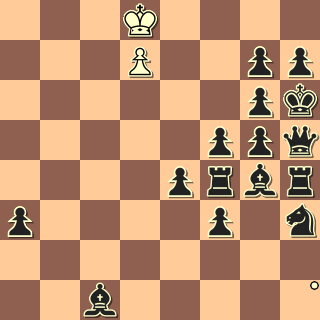# power and powerdifference

The power of a piece is its material value: 1 for a pawn; 3 for a bishop or knight; 5 for a rook; and 9 for a queen. Kings and empty squares are ignored, or nominally have zero power.

The power of a set of squares in a position is the sum of the power of all the pieces on squares in that set in the position.

## power filter

The `power` power filter is the word `power` followed by a range followed by a set filter:
`      power filter := 'power' range set-filter`
For example,
`      power 15 1000 A`
is a power filter with range `15 1000` and set filter of `A.`

A power filter matches a position if the power of the pieces on the squares denoted by its set filter in that position lie within its range. Thus, `power 15 1000 A` matches positions where the power (material value) of the White pieces is at least 15.

V. Kosek
1.p Slovensky Narod
1926```result 1-0
event "1.p"
initial
power 7 [Aa]```

The diagram above shows the earliest first prize win study with an initial total power of 7.

## powerdifference

A powerdifference filter is the word `powerdifference` followed by a range and an argument list with two set filters:
`      powerdifference := 'powerdifference' range '(' setfilter1 setfilter2 ')'`
A powerdifference filter matches a position if the difference in the powers of its first and second arguments lies within the range. For example:

T. Dawson
Schackvarlden
1945```result 1-0
wtm
not check
power 1 A
powerdifference -1000 -20 (A a)```
The above study by Dawson has a powerdifference of 35.
Note that the range can include negative values.

Examples